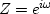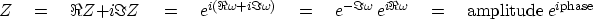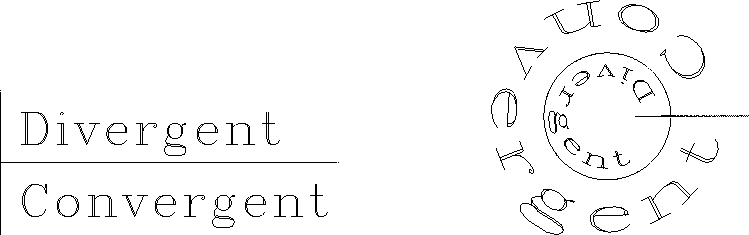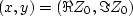Next: The meaning of divergence Up: INSTABILITY Previous: The unit circle

## The mapping between Z and complex frequency

We are familiar with the fact that real values ofcorrespond to complex values of.Now let us look at complex values of:(44)
Thus, when, |Z|<1. In words, we transform the upper half of the-plane to the interior of the unit circle in the Z-plane. Likewise, the stable region for poles is the lower half of the-plane, which is the exterior of the unit circle. Figure 12 shows the transformation. Some engineering books choose a different sign convention (), but I selected the sign convention of physics.Z
Figure 12
Left is the complex-plane with axes. Right is the Z-plane with axes. The words Convergent'' and Divergent'' are transformed by.Next: The meaning of divergence Up: INSTABILITY Previous: The unit circle
Stanford Exploration Project
10/21/1998